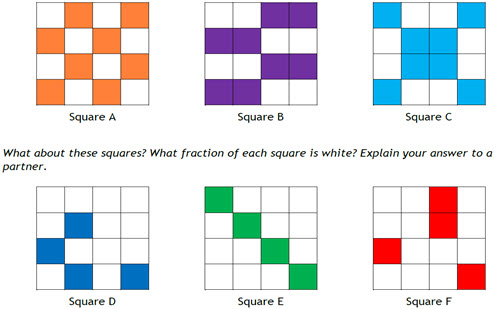Go to website

# reSolve: Authentic Problems: Expanded Square

This sequence of four lessons explores concepts around informal area and symmetry. Students design an 'expanded square' where approximately half the area of the original square is flipped to the outside. The lessons provide opportunities for students to devise and use methods to informally measure area, record their mathematical thinking and justify their designs. Each lesson is outlined in detail including curriculum links, vocabulary, materials needed, sample answers, discussion points and student resources. This sequence is part of the reSolve: Mathematics by Inquiry special topic 'Authentic Problems'.

Year level(s) Year 4
Audience Teacher
Purpose Teaching resource
Teaching strategies and pedagogical approaches Mathematics investigation
Keywords area, symmetry, equivalent fractions, maths investigations, irregular polygons

## Curriculum alignment

Curriculum connections Numeracy
Strand and focus Number, Measurement, Build understanding, Apply understanding, Space
Topics Fractions, Shapes and objects, Area, volume and surface area
AC: Mathematics (V9.0) content descriptions
AC9M4M02
Recognise ways of measuring and approximating the perimeter and area of shapes and enclosed spaces, using appropriate formal and informal units

AC9M4N03
Find equivalent representations of fractions using related denominators and make connections between fractions and decimal notation

AC9M4SP03
Recognise line and rotational symmetry of shapes and create symmetrical patterns and pictures, using dynamic geometric software where appropriate

Numeracy progression Understanding units of measurement (P6)
Interpreting fractions (P5)
Understanding geometric properties (P4)